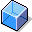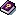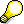Material Cost Estimate vs. Preliminary Order Cost EstimateDefinition

Report that compares a material cost estimate with thepreliminary cost estimate for a manufacturing order.

Use

You can use this report to compare a material cost estimate with the planned costs of manufacturing orders for this material.

You can find this report in the following application components of Product Cost Controlling:

• Product Cost Planning
• Product Cost by Order

Structure

The view of a cost estimate relevant to inventory accounts is compared with a preliminary order cost estimate on the basis of original cost elements. The cost element itemization of material costing is derived with the following strategy:

1. The system reads the cost element itemization of the cost estimate, if it exists.In the SAP standard system, you can no longer control whether a cost element itemization is saved. The cost element itemization is always generated dynamically on the basis of the itemization.

1. A cost element itemization is generated dynamically from the itemization of the cost estimate. This dynamically generated cost element itemization is not saved in the system at any time.

The cost base is calculated as follows:

• If you specify a lot size, both the values of the material cost estimate and the values of the preliminary order cost estimate are converted to that lot size.
• If you don’t specify a lot size, the system will convert the values to the costing lot size of the material cost estimate.Because the system cannot convert if the units of measure are different, this comparison is normally only suitable for a material cost estimate with the orders for that material.